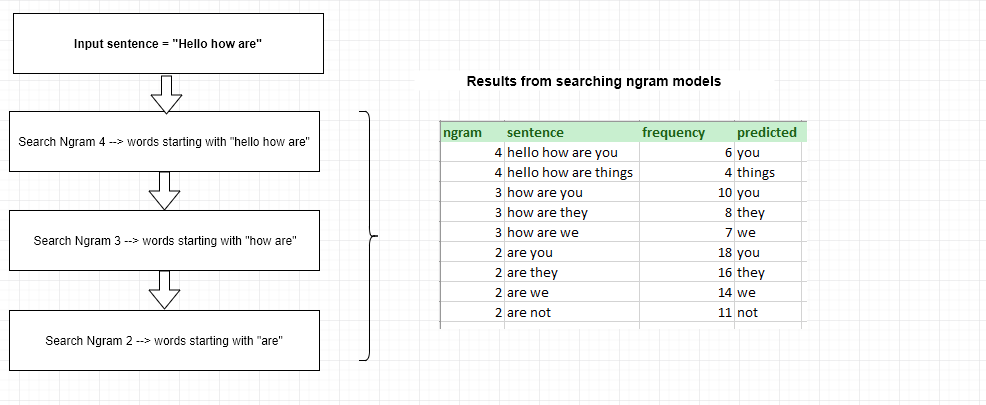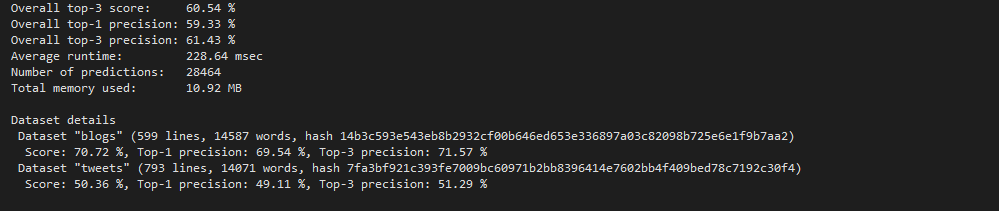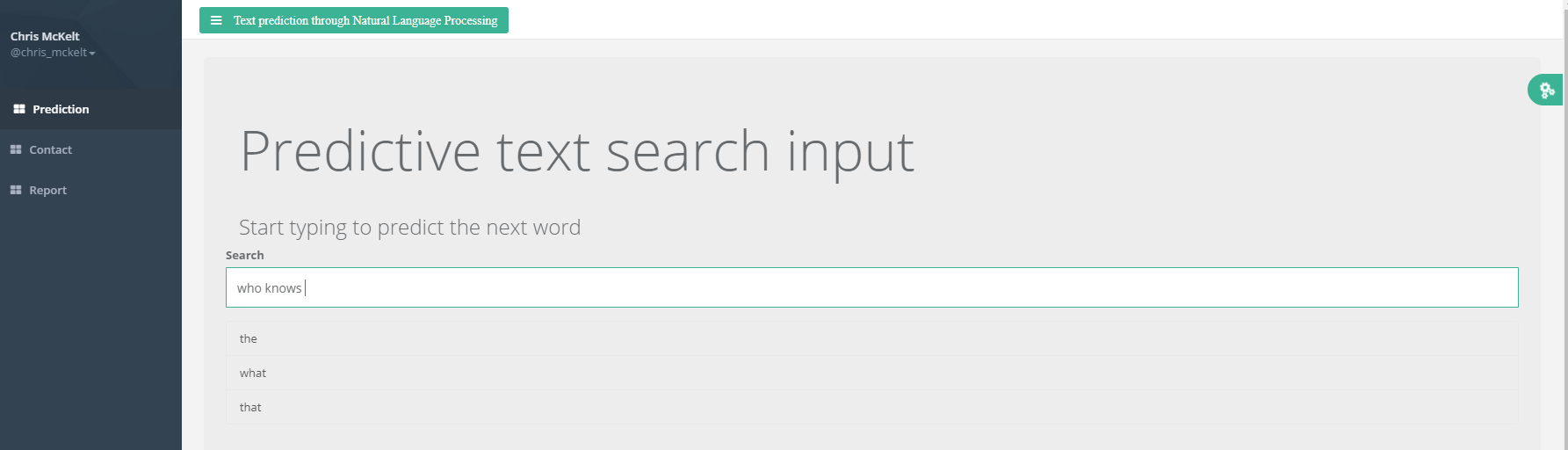## Introduction

This report outlines the methodology for building a word prediction application using Natural Language Processing techniques as part of the the Coursera Data Science Specialization.

The milestone report outlines the initial approach to building a series of ngram models from a range of text documents.

## Prediction models

• A cleaned corpus sample was used to create 5 bag of word ‘ngram’ models
• The below process was used to search for words from an input sentence## Algorithm used to make the prediction

A Stupid Backoff smoothing strategy was used to calculate a ‘score’ for each word follows:

if the rows ngram model was 5
score = matched 5 gram Count / input 4 gram Count
else if the rows ngram model was 4
score = 0.4 * matched 4 gram Count / input 3 gram Count
else if the rows ngram model was 3
score = 0.4 * 0.4 * matched 3 gram Count / input 2 gram Count
else if the rows ngram model was 2
score = 0.4 * 0.4 * 0.4 * matched 2 gram Count / input 1 gram Count
ngram predicted score
4 you 0.2
3 you 0.1
2 you 0.05
The total score for the predicted word ‘you’ is (0.2 + 0.1 + 0.05) = 0.35 The final scoring is aggregated and summed for each word. The top 3 words are selected according to the highest score.

## Evaluation

The prediction model was evaluated using the Benchmark.R tool (see references for source).

Initial predicts were quite high but also quite slow. The decision to only use 1-3 ngram models sped up the search time by half but also dropped the accuracy by 10%.## Instructions

To use the application navigate to the following URL

https://chrismckelt.shinyapps.io/datascience-capstone/

To use the application start typing in text.When no results are found the 3 most common words from the English language (‘the’, ‘be’, ‘to’) are returned as a response.

Click on the green side menu for visual display options.